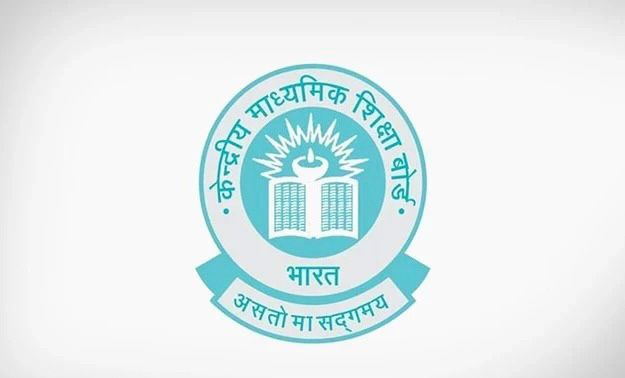# The greatest number which when divides 1251, 9377 and 15628 learn remainder 1, 2 and 3 respectively is

CBSE Maths Exam 2021-22 Term 1, question: The greatest number which when divides 1251, 9377 and 15628 learn remainder 1, 2 and 3 respectively isa) 575

b) 450

c) 750

d) 625